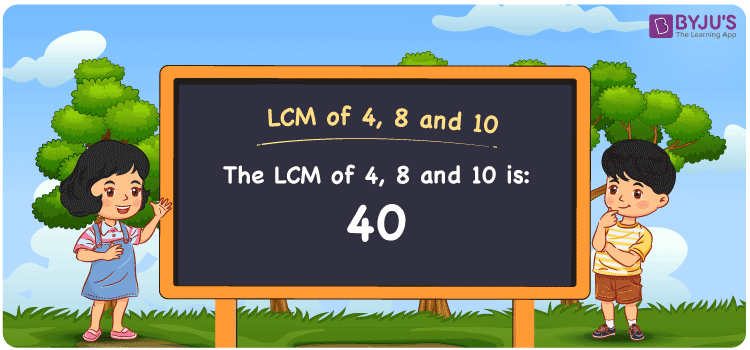# LCM of 4 8 and 10

LCM of 4, 8 and 10 is 40. LCM stands for Least Common Multiple or the Lowest common multiple. It is also referred to as LCD Least Common Divisor. It is the positive integer that is a common multiple to the given set of numbers. Here the given set of numbers are 4, 8 and 10. The smallest common multiple for all these numbers among many is 40, and hence it is the LCM. You can refer to LCM with Examples to understand better.

## What is LCM of 4, 8 and 10

The Least Common Multiple or Lowest Common Multiple of 4, 8 and 10 is 40.## How to Find LCM of 4, 8 and 10?

LCM of 4, 8 and 10 can be determined using three methods:

• Prime Factorisation
• Division method
• Listing the multiples

### LCM of 4, 8 and 10 Using Prime Factorisation Method

In the Prime Factorisation method, the numbers 4, 8 and 10 can be expressed as;

4 = 2 × 2

8 = 2 × 2 × 2

10 = 2 × 5

2 is the only common prime factor for all 3 numbers. This is multiplied by the other factors of 4, 8 and 10 to get the LCM.

LCM(4, 8 and 10) = 2 × 2 × 2 × 5 = 40

### LCM of 4, 8 and 10 Using Division Method

In the Division Method, the given numbers 4, 8 and 10 are divided together with prime divisors. The product of prime divisors forms the LCM.

 2 4 8 10 2 2 4 5 2 1 2 5 5 1 1 5 × 1 1 1

LCM(4, 8 and 10) = 2 × 2 × 2 × 5 = 40

### LCM of 4, 8 and 10 Using Listing the Multiples

By listing all the multiples of 4, 8 and 10, we can identify the LCM. Below is the list of multiples for 4, 8 and 10

 Multiple of 4 Multiple of 8 Multiple of 10 4 8 10 8 16 20 12 24 30 16 32 40 20 40 50 24 48 60 28 56 70 32 64 80 36 72 90 40 80 100

LCM(4, 8 and 10) = 40

## Video Lesson on Applications of LCM## Solved Examples

What is the smallest number that is divisible by 4, 8 and 10?

Answer: 40 is the smallest number that is divisible by 4, 8 and 10.

What is the LCM of 2, 5 and 40?

Answer: LCM of 2, 5 and 40 is 40.

## Frequently Asked Questions on LCM of 4, 8 and 10

### What is the LCM of 4, 8 and 10?

The LCM of 4, 8 and 10 is 40.

### What is the difference between the LCM 4, 8 and 10 and HCF of 4, 8 and 10?

The LCM of 4, 8 and 10 is 40 and HCF of 4, 8 and 10 is 2.

### Is the LCM and LCD both the same for 4, 8 and 10?

Yes. The LCM and Lowest Common Divisor are both the same for 4, 8 and 10  which is 40.

### What are the first 3 common multiples of 4, 8 and 10?

The first three common multiples of 4, 8 and 10 are 40, 80, and 120.

### Is the LCM of 4 and 8 the same as the LCM of 8 and 10?

No. LCM of 4 and 8 is 8, whereas the LCM of 8 and 10 is 40. They are not the same.